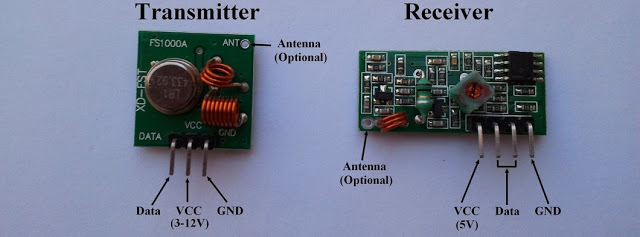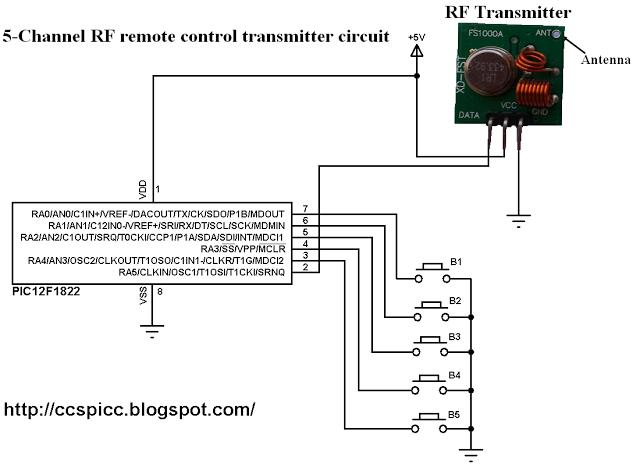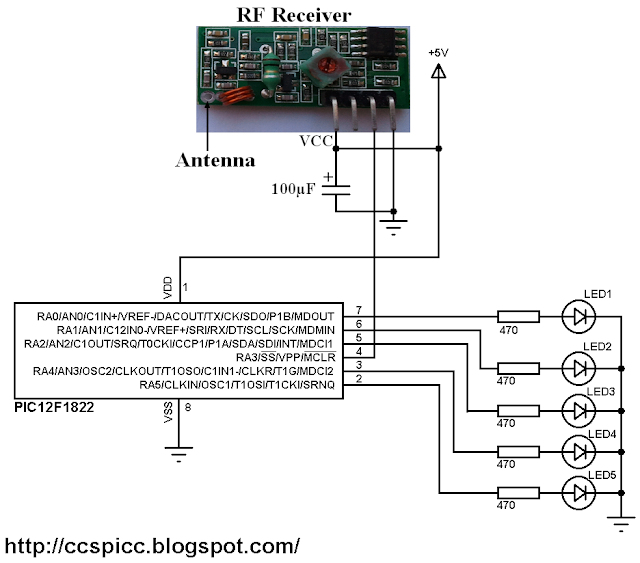433MHz RF Remote control system with PIC microcontroller

This project shows how to use low cost 433MHz RF transmitter/receiver modules to build a 5-channel wireless RF remote control system using 2 x PIC12F1822 microcontrollers.
The used RF modules in this project are cheap and easy to use with any microcontroller. These modules are shown below with pin configuration:Communication protocol:
In this 433MHz RF project data is transmitted from the transmitter circuit to the receiver circuit using NEC protocol. The NEC protocol uses pulse distance encoding of the bits. Each pulse is a 562.5µs long.
Logic 0: 562.5µs pulse burst followed by a 562.5µs space, with a total transmit time of 1125µs (562.5 x 2).
Logic 1: a 562.5µs pulse burst followed by a 1687.5µs (562.5 x 3) space, with a total transmit time of 2250µs (562.5 x 4).
The following topic shows how to decode an IR remote control uses the NEC communication protocol:
Extended NEC Protocol Decoder Using PIC12F1822 Microcontroller

Components List:
RF Transmitter Circuit:

• PIC12F1822 Microcontroller
• 433MHz or 315MHz RF Transmitter
• 5 Push Buttons
• +5V Power Source
• Protoboard
• Jumper Wires

• PIC12F1822 Microcontroller
• 433MHz or 315MHz RF Receiver
•  100uf Capacitor
• 5 LEDs
• 5 x 470 Ohm Resistors
• +5V Power Source
• Protoboard
• Jumper Wires

5-Channel 433MHz RF remote control transmitter circuit:
The RF transmitter circuit schematic is shown below.In the circuit there 5 push buttons and each button sends a different RF signal code. The RF transmitter is the element that sends the RF signals to the RF receiver circuit.
PIC12F1822 internal pull-ups are enabled by the software and the internal oscillator is used as usual.

5-Channel 433MHz RF remote control transmitter using PIC12F1822 CCS PIC C code:
In the circuit there are 5 push buttons connected to RA0, RA1, RA2, RA3 and RA4 which means that pins must be configured as inputs and the RA5 pin as output.
Internal pull-ups are enabled for the input pins with the following line:
port_a_pullups(0x1F);
The five buttons transmit the following signals respectively:
0x40BF00FF — 0x40BF807F — 0x40BF40BF — 0x40BF20DF — 0x40BFA05F
1s and 0s are transmitted as:
Transmit 1 : output_high(PIN_A5);
Transmit 0: output_low(PIN_A5);
For example the transmission of 9ms pulse and 4.5ms space:
// Send 9ms pulse
output_high(PIN_A5);
delay_ms(9);
// Send 4.5ms space
output_low(PIN_A5);
delay_us(4500);

CCS C code:

```// NEC protocol 433MHz RF remote control transmitter using PIC12F1822 CCS PIC C code
// http://ccspicc.blogspot.com/
// [email protected]
// Use at your own risk

#include <12F1822.h>
#fuses NOMCLR INTRC_IO PLL_SW
#use delay(clock=32000000)
#use fast_io(A)

void send_signal(unsigned int32 number){
int8 i;
// Send 9ms pulse
output_high(PIN_A5);
delay_ms(9);
// Send 4.5ms space
output_low(PIN_A5);
delay_us(4500);
// Send data
for(i = 0; i < 32; i++){
// If bit is 1 send 560us pulse and 1680us space
if(bit_test(number, 31 - i)){
output_high(PIN_A5);
delay_us(560);
output_low(PIN_A5);
delay_us(1680);
}
// If bit is 0 send 560us pulse and 560us space
else{
output_high(PIN_A5);
delay_us(560);
output_low(PIN_A5);
delay_us(560);
}
}
// Send end bit
output_high(PIN_A5);
delay_us(560);
output_low(PIN_A5);
delay_us(560);
}
void main() {
setup_oscillator(OSC_8MHZ | OSC_PLL_ON);            // Set internal oscillator to 32MHz (8MHz and PLL)
output_a(0);
set_tris_a(0x1F);                                   // Configure RA5 pin as output and others as inputs
port_a_pullups(0x1F);                               // Enable internal pull-ups for pins RA0,RA1,RA2,RA3 & RA4
while(TRUE){
while(!input(PIN_A0)){
send_signal(0x40BF00FF);
delay_ms(500);
}
while(!input(PIN_A1)){
send_signal(0x40BF807F);
delay_ms(500);
}
while(!input(PIN_A2)){
send_signal(0x40BF40BF);
delay_ms(500);
}
while(!input(PIN_A3)){
send_signal(0x40BF20DF);
delay_ms(500);
}
while(!input(PIN_A4)){
send_signal(0x40BFA05F);
delay_ms(500);
}
}
}```

5-Channel 433MHz RF remote control receiver circuit:In the 433MHz RF receiver circuit there are 5 LEDs and an RF receiver. The RF receiver receives radio signals transmitted from the RF transmitter. Each LED is remotely controlled from one button in the transmitter circuit.
PIC12F1822 internal oscillator is used and MCLR pin is configured as a digital input pin.
5-Channel 433MHz RF remote control receiver using PIC12F1822 CCS PIC C code:
As in the RF transmitter the internal oscillator of the RF receiver microcontroller is used.
Timer1 is configured to increment every 1us and it is used to measure pulses and spaces duration (@ 32MHz mcu frequency).
SETUP_TIMER_1(T1_INTERNAL | T1_DIV_BY_8);
For example to check the 9ms pulse the following lines are used:
// Check 9ms pulse (remote control sends logic high)
SET_TIMER1(0);
while(input(IR_Sensor) && (count < 9500))
count = GET_TIMER1();
if( (count > 9499) || (count < 8500))
return FALSE;

And checking the 4.5ms space as follows:
// Check 4.5ms space (remote control sends logic low)
SET_TIMER1(0);
count = 0;
while((!input(IR_Sensor)) && (count < 5000))
count = GET_TIMER1();
if( (count > 4999) || (count < 4000))
return FALSE;

Full Code:

```// NEC protocol 433MHz RF remote control receiver using PIC12F1822 CCS PIC C code
// http://ccspicc.blogspot.com/
// [email protected]
// Use at your own risk

#include <12F1822.h>
#fuses NOMCLR INTRC_IO PLL_SW
#use delay(clock=32000000)
#use fast_io(A)

unsigned int32 rf_code;
unsigned int16 count = 0;
unsigned int8 i;
// Check 9ms pulse (RF remote control sends logic high)
SET_TIMER1(0);
count = GET_TIMER1();
if( (count > 9499) || (count < 8500))
return FALSE;
// Check 4.5ms space (RF remote control sends logic low)
SET_TIMER1(0);
count = 0;
count = GET_TIMER1();
if( (count > 4999) || (count < 4000))
return FALSE;
// Read RF message (32 bits)
for(i = 0; i < 32; i++){
SET_TIMER1(0);
count = 0;
count = GET_TIMER1();
if( (count > 649) || (count < 500))
return FALSE;
count = 0;
SET_TIMER1(0);
count = GET_TIMER1();
if( (count > 1799) || (count < 400))
return FALSE;
if( count > 1000)                                 // If space width > 1ms
bit_set(rf_code, (31 - i));                     // Write 1 to bit (31 - i)
else                                              // If space width < 1ms
bit_clear(rf_code, (31 - i));                   // Write 0 to bit (31 - i)
}
return TRUE;
}
void main() {
setup_oscillator(OSC_8MHZ | OSC_PLL_ON);            // Set internal oscillator to 32MHz (8MHz and PLL)
output_a(0);
set_tris_a(8);
SETUP_TIMER_1(T1_INTERNAL | T1_DIV_BY_8);
while(TRUE){
if(rf_code == 0x40BF00FF)
output_toggle(PIN_A0);
if(rf_code == 0x40BF807F)
output_toggle(PIN_A1);
if(rf_code == 0x40BF40BF)
output_toggle(PIN_A2);
if(rf_code == 0x40BF20DF)
output_toggle(PIN_A4);
if(rf_code == 0x40BFA05F)
output_toggle(PIN_A5);
}
}
}```

#### Current Project / Post can also be found using:

• pic microcontroller with wifi